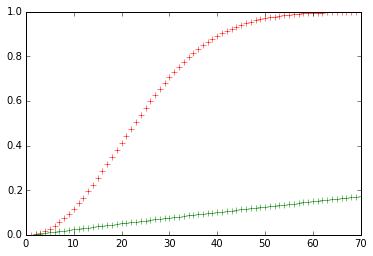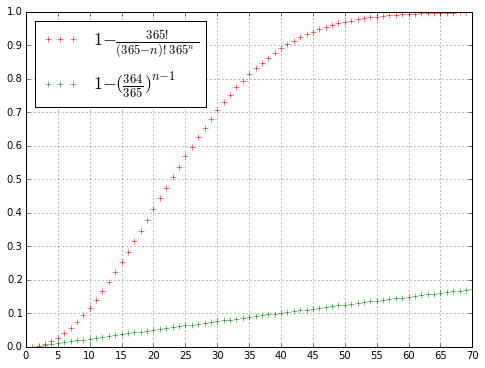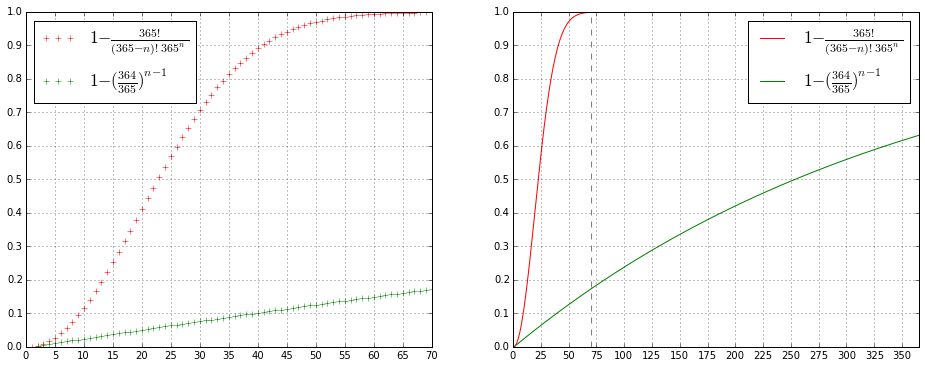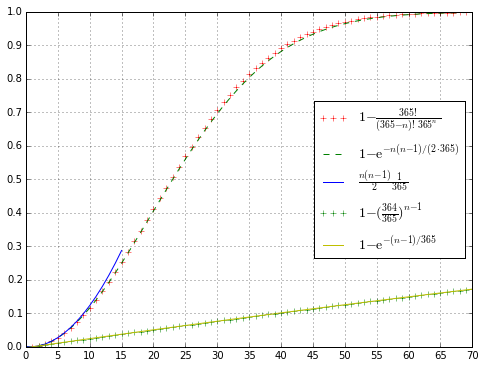In :
%pylab inline

Populating the interactive namespace from numpy and matplotlib

In :
from scipy.misc import factorial,comb
factorial(10)/(factorial(6)*factorial(4)), comb(10,4,exact=True)

Out:
(210.0, 210)

While the above functions could be used, recall that arange(365,365-70,-1) gives an array of the seventy numbers from 365 down to 296, so arange(365,365-70,-1)/365. gives an array of the successive fractions of not having a coincident birthday, and 1-cumprod(arange(365,365-70,-1)/365.) then gives the probability of at least one coincidence for numbers of people from 1 through 70.
Similarly, ones(69)/365. is an array of 69 copies of 1/365. so cumprod(ones(69)*364./365.) gives successive powers of 1/365., and 1 minus that gives the probability of coincidence with a given specific person for numbers of people from 2 through 70. These two functions can be plotted as follows:

In :
plot(range(1,71),1-cumprod(arange(365,365-70,-1)/365.),'r+')
plot(range(2,71),1-cumprod(ones(69)*364./365.),'g+');We can make the graph a bit larger, and add a grid, to see that the probability of coincident birthdays exceeds .5 starting at n equal to 23, is about .97 at n equal to 50, and is virtually guaranteed by n equal to 70:

In :
figure(figsize=(8,6))
plot(range(1,71),1-cumprod(arange(365,295,-1)/365.),'r+')
plot(range(2,71),1-cumprod(ones(69)*364./365.),'g+')
yticks(arange(0,1.1,.1));
xlim(0,70)
xticks(range(0,71,5))
grid('on')
legend([r'$1-\frac{365!}{(365-n)!\ 365^n}$',
r'$1-(\frac{364}{365})^{n-1}$'],
numpoints=3,loc='upper left',fontsize=18);We can also expand the range to see what happens to the second curve when we reach n equal to 365, by plotting

plot(range(1,366),1-cumprod(arange(365,0,-1)/365.),'r')
plot(range(2,366),1-cumprod(ones(364)*364./365.),'g')



Or we can define a function, and use subplot to put the two figures side by side. At n equal to 365, the lower curve has a probability of roughly .63:

In :
def bdayplot(n,inc=25,sym='',loc=None,):
plot(range(1,n+1),1-cumprod(arange(365,365-n,-1)/365.),'r'+sym)
plot(range(2,n+1),1-cumprod(ones(n-1)*364./365.),'g'+sym)
xticks(range(0,n+1,inc))
yticks(arange(0,1.1,.1))
xlim(0,n)
grid('on')
legend([r'$1-\frac{365!}{(365-n)!\ 365^n}$',
r'$1-(\frac{364}{365})^{n-1}$'],
numpoints=3,loc=loc,fontsize=17);

figure(figsize=(16,6))
subplot(1,2,1)
bdayplot(70,5,'+','upper left')
subplot(1,2,2)
bdayplot(365)
plot([70,70],[0,1],c='gray',linestyle='--');Note that $\frac{n(n-1)}{2}\frac{1}{365}$ is a good approximation to the first function (red) for small $n$, up to about $n=14$ (it just counts the number of pairs times the probability that a pair has coincident birthdays, so works as long as the pairs are approximately independent).
An even better approximation is given by $1-{\rm e}^{-n(n-1)/(2\cdot365)}$, which works extremely well throughout the full range of $n$ (dotted green line below). (One way of deriving this is to use Stirling's approximation for the factorial: $n! \sim n^n{\rm e}^{-n}\sqrt{2\pi n}$ for large $n$.)

Note also that $1-{\rm e}^{-(n-1)/365}$ is a good approximation to the second function (green) for all $n$, hence the value $1-{\rm e}^{-1}\approx.63$ for $n=366$ in the above plot.

In :
figure(figsize=(8,6))
plot(range(1,71),1-cumprod(arange(365,365-70,-1)/365.),'r+')
xlim(0,70)
ylim(0,1)
grid('on')
plot(range(70),[1-exp(-n*(n-1)/(2.*365)) for n in range(70)],'g--')
plot([n*(n-1)/(2.*365) for n in range(16)],'b')
plot(range(2,71),1-cumprod(ones(69)*364./365.),'g+')
plot([1-exp(-(n-1)/365.) for n in range(366)],'y')
xticks(range(0,75,5))
yticks(arange(0,1.1,.1));
legend([r'$1-\frac{365!}{(365-n)!\ 365^n}$',
r'$1-{\rm e}^{-n(n-1)/(2\cdot365)}$',
r'$\frac{n(n-1)}{2}\frac{1}{365}$',
r'$1-(\frac{364}{365})^{n-1}$',
r'$1-{\rm e}^{-(n-1)/365}$'],
numpoints=3,loc='center right',fontsize=14);In [ ]: